Select Page

Are you afraid that AI might take your job? Make sure you are the one who is building it.

STAY RELEVANT IN THE RISING AI INDUSTRY! 🖖

Don’t get me wrong, feature engineering is not there just to optimize models. Sometimes we need to apply these techniques so our data is compatible with the machine learning algorithm. Machine learning algorithms sometimes expect data formatted in a certain way, and that is where feature engineering can help us. Apart from that, it is important to note that data scientists and engineers spend most of their time on data preprocessing. That is why it is important to master these techniques. In this article we explore:

## Dataset & Prerequisites

For the purpose of this tutorial, make sure that you have installed the following Python libraries:

• NumPy – Follow this guide if you need help with installation.
• SciKit Learn – Follow this guide if you need help with installation.
• Pandas – Follow this guide if you need help with installation.
• Matplotlib – Follow this guide if you need help with installation.
• SeaBorn – Follow this guide if you need help with installation.

Once installed make sure that you have imported all the necessary modules that are used in this tutorial.Data that we use in this article is from PalmerPenguins Dataset. This dataset has been recently introduced as an alternative to the famous Iris dataset. It is created by Dr. Kristen Gorman and the Palmer Station, Antarctica LTER. You can obtain this dataset here, or via Kaggle. This dataset is essentially composed of two datasets, each containing data of 344 penguins. Just like in Iris dataset there are 3 different species of penguins coming from 3 islands in the Palmer Archipelago.

Also, these datasets contain culmen dimensions for each species. The culmen is the upper ridge of a bird’s bill. In the simplified penguin’s data, culmen length and depth are renamed as variables culmen_length_mm and culmen_depth_mm. Loading this dataset is done using Pandas: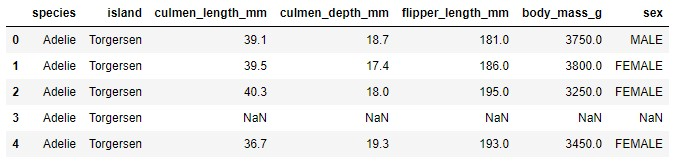## 1. Imputation

Data that we get from clients can come in all shapes and forms. Often it is sparse, meaning some samples may miss data for some features. We need to detect those instances and remove those samples or replace empty values with something. Depending on the rest of the dataset, we may apply different strategies for replacing those missing values. For example, we may fill these empty slots with average feature value, or maximal feature value. However, let’s first detect missing data. For that we can use Pandas:

This means that there are instances in our dataset that are missing values in some of the features. There are two instances that are missing the culmen_length_mm feature value and 10 instances that are missing the sex feature. We were able to see that even in the first couple of samples (NaN means Not a Number, meaning missing value):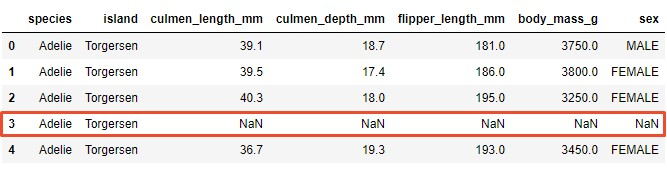The easiest deal with missing values is to drop samples with missing values from the dataset, in fact, some machine learning platforms automatically do that for you. However, this may reduce the performance of the dataset, because of the reduced dataset. The easy way to do it is again using Pandas:Note that the third sample with missing values is removed from the dataset. This is not optimal, but sometimes it is necessary since most of the machine learning algorithms don’t work with sparse data. The other way is to use imputation, meaning to replace missing values. To do so we can pick some value, or use the mean value of the feature, or an average value of the feature, etc. Still, we need need to be careful. Observe missing value at the row with index 3:If just replace it with simple value, we apply the same value for categorical and for numerical features: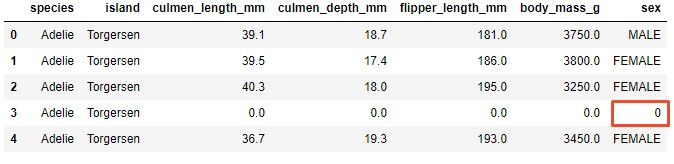This is not good. So, here is the proper way. We detected missing data in numerical features culmen_length_mm, culmen_depth_mm, flipper_length_mm and body_mass_g. For the imputation value of these features, we will use the mean value of the feature. For the categorical feature ‘sex‘, we use the most frequent value. Here is how we do it:

Observe how the mentioned third sample looks like now: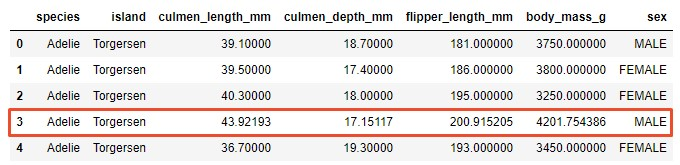Often, data is not missing, but it has an invalid value. For example, we know that for the ‘sex‘ feature we can have two values: FEMALE and MALE. We can check if we have values other than this:As it turnes out we have one record that has value ‘.’ for this feature, which is not correct. We can observe these instances as a missing data and drop them or replace them:

## 2. Categorical Encoding

One way to improve your predictions is by applying clever ways when working with categorical variables. These variables, as the name suggests, have discrete values and represent some sort of category or class. For example color can be categorical variable (‘red’, ‘blue‘, ‘green‘). The challenge is including these variables into data analysis and use them with machine learning algorithms. Some machine learning algorithms support categorical variables without further manipulation, but some don’t. That is why we use a categorical encoding. In this tutorial, we cover several types of categorical encoding, but before we continue, let’s extract those variables from our dataset into a separate variable and mark them as categorical type:Ok, now we are ready to roll. We start with the simplest form of encoding Label Encoding.

### 2.1 Label Encoding

Label encoding is converting each categorical value into some number. For example, the ‘species‘ feature contains 3 categories. We can assign value 0 to Adelie, 1 to Gentoo and 2 to Chinstrap. To perform this technique we can use Pandas:As you can see, we added three new features each containing encoded categorical features. From the first five instances, we can see that species category Adelie is encoded with value 0, island category Torgensesn is encoded with value and sex categories FEMALE and MALE are encoded with values 0 and 1 respectively.

### 2.2 One-Hot Encoding

This is one of the most popular categorical encoding techniques. It spreads the values in a feature to multiple flag features and assigns values 0 or 1 to them. This binary value represents the relationship between non-encoded and encoded features.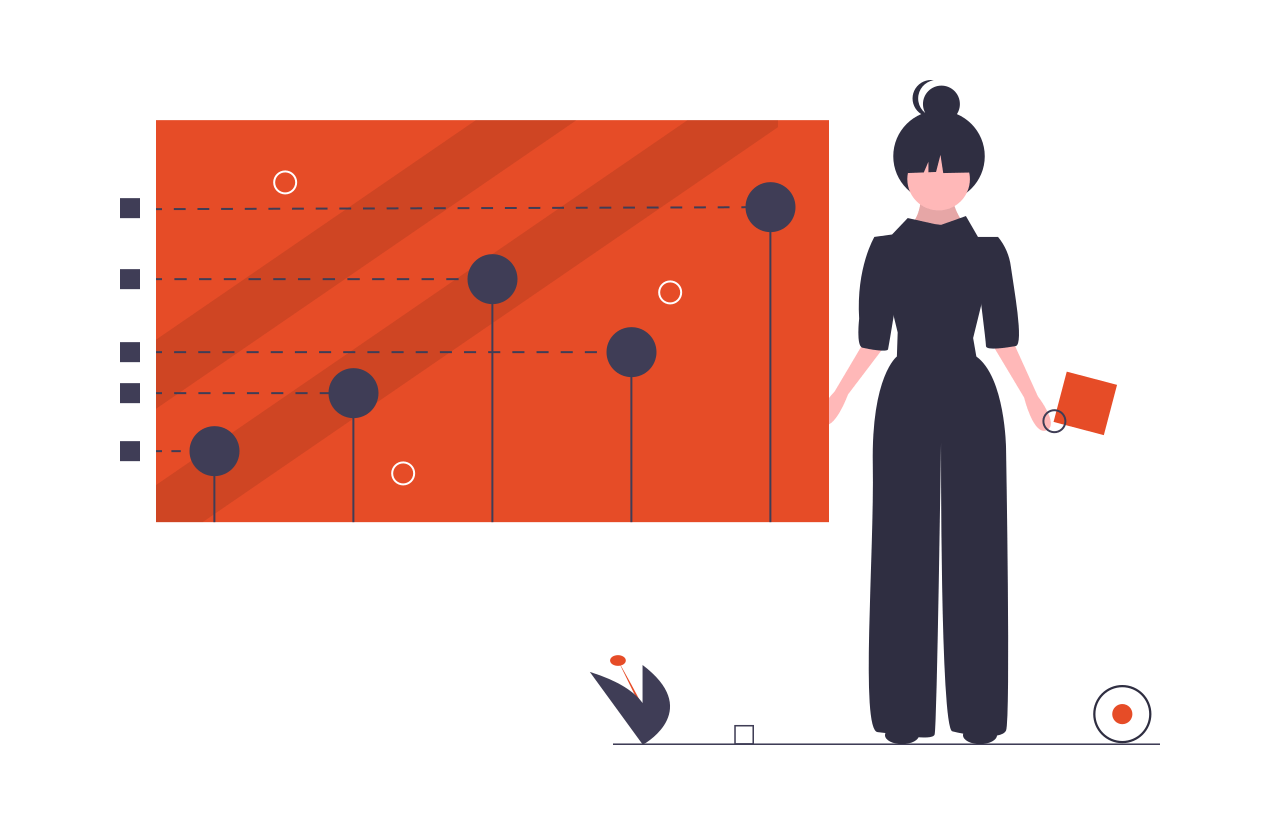For example, in our dataset, we have two possible values in ‘sex‘ feature: FEMALE and MALE. This technique will create two separate features labeled let’s say ‘sex_female‘ and ‘sex_male‘. If in the ‘sex‘ feature we have value ‘FEMALE‘ for some sample, the ‘sex_female‘ will be assigned value 1 and ‘sex_male‘ will be assigned value 0. In the same way, if in the ‘sex‘ feature we have the value ‘MALE‘ for some sample, the ‘sex_male‘ will be assigned value 1 and ‘sex_female‘ will be assigned value 0. Let’s apply this technique to our categorical data and see what we get:As you we gave some new columns there. Essentially, every category in each feature got a separate column. Often, just one-hot encoded values are used as input to a machine learning algorithm.

### 2.3 Count Encoding

Count encoding is converting each categorical value to its frequency, ie. the number of times it appears in the dataset. For example, if the ‘species‘ feature contains 6 occurrences of class Adelie we will replace every Adelie value with the number 6. Here is how we do that in the code: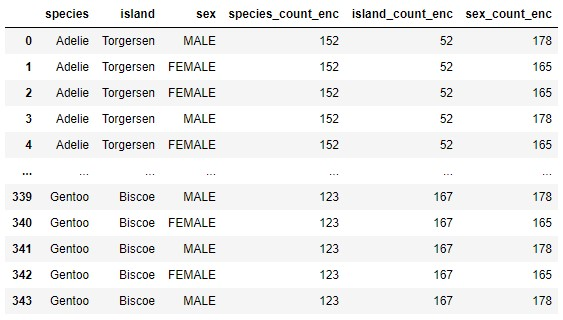Notice how every category value is replaced with the number of occurances.

### 2.4 Target Encoding

Unlike previous techniques, this one is a little bit more complicated. It replaces a categorical value with the average value of the output (ie. target) for that value of the feature. Essentially, all you need to do is calculate the average output for all the rows with specific category value. Now, this is quite straight forward when the output value is numerical. If the output is categorical, like in our PalmerPenguins dataset, we need to apply some of the previous techniques to it.Often this average value is blended with the outcome probability over the entire dataset in order to reduce the variance of values with few occurrences.  It is important to note that since category values are calculated based on the output value, these calculations should be done on the training dataset and then applied to other datasets. Otherwise, we would face information leakage, meaning that we would include information about the output values from the test set inside of the training set. This would render our tests invalid or give us false confidence. Ok, let’s see how we can do this in code:

Here we used label encoding for output feature and then calculated mean values for categorical features ‘island‘ and ‘sex‘. Here is what we get for the ‘island‘ feature:

This means that values Biscoe, Dream and Torgersen will be replaced with values 1.473054, 0.548387 and 0 respectively. For the ‘sex‘ feature we have a similar situation:

Meaning that values FEMALE and MALE will be replaced with 0.909091 and 0.921348 respectively. Here is what that looks like in the dataset: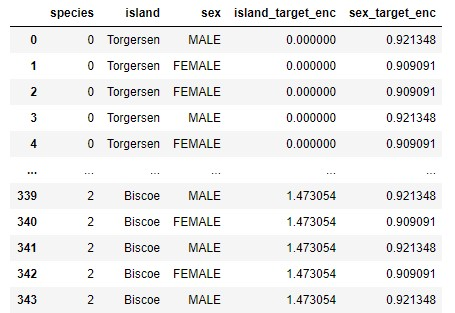### 2.5 Leave One Out Target Encoding

The final type of encoding that we explore in this tutorial is built on top of Target Encoding. It works in the same way as Target encoding with one difference. When we are calculating the mean output value for the sample, we exclude that sample. Here is how it is done in the code. First, we define a function that does this:

And then we apply it to categorical values in our dataset:## 3. Handling Outliers

Outliers are values that are deviating from the whole distribution of the data. Sometimes these values are mistakes and wrong measurements and should be removed from datasets, but sometimes they are valuable edge-case information. This means that sometimes we want to leave these values in the dataset, since they may carry some important information, while other times we want to remove those samples, because of the wrong information.

In a nutshell, we can use the Inter-quartile range to detect these points. Inter-quartile range or IQR indicates where 50 percent of data is located. When we are looking for this value we first look for the median, since it splits data into half. Then we are locating the median of the lower end of the data (denoted as Q1) and the median of the higher end of the data (denoted as Q3). Data between Q1 and Q3 is the IQR. Outliers are defined as samples that fall below Q11.5(IQR) or above Q3 + 1.5(IQR). We can do this using a boxplot. The purpose of the boxplot is to visualize the distribution. In essence, it includes important points: max value, min value, median, and two IQR points (Q1, Q3). Here is how one example of a boxplot looks like: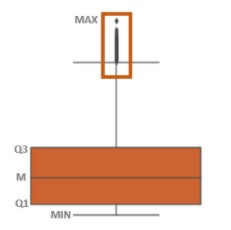Let’s apply it to PalmerPenguins dataset: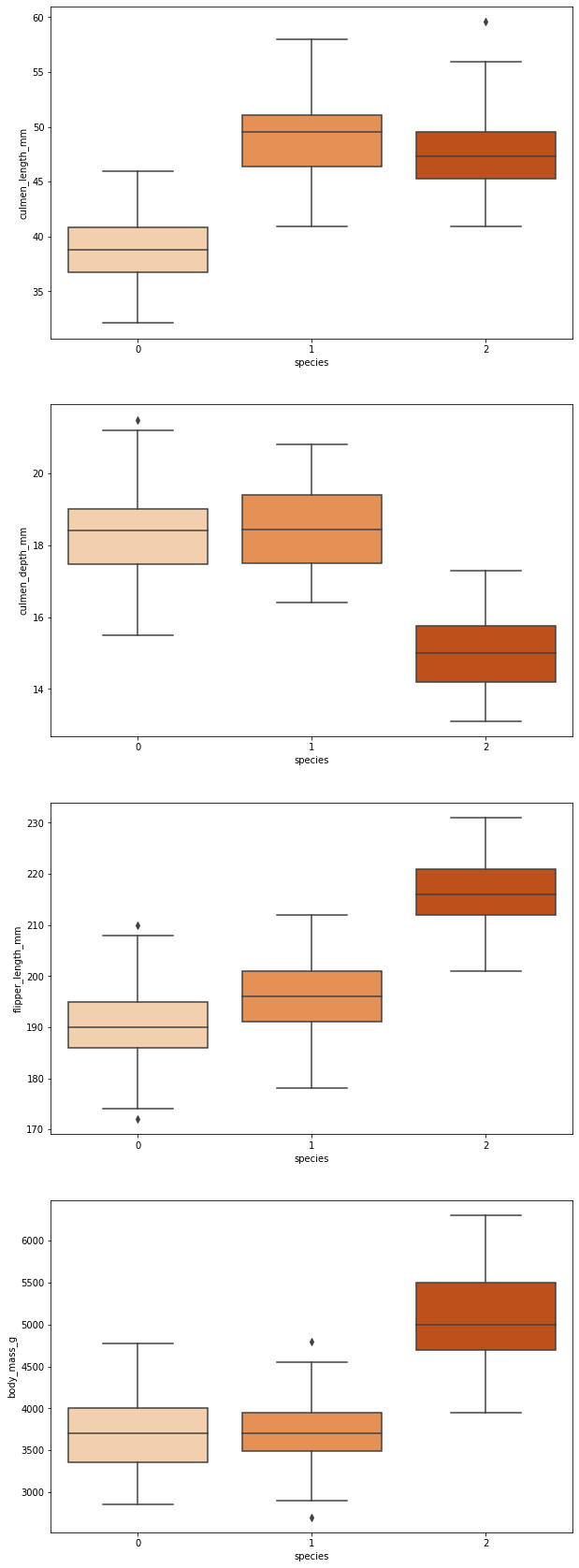The other way for detecting and removing outliers would by using standard deviation.Note that now we have only 100 samples left after this operation. Here we need to define the factor by which we multiply the standard deviation. Usually, we use values between 2 and 4 for this purpose.

Finally, we can use a method to detect outliers is to use percentiles. We can assume a certain percentage of the value from the top or the bottom as an outlier. Again a value for the percentiles we use as outliers border depends on the distribution of the data. Here is what we can do on PalmerPenguins dataset: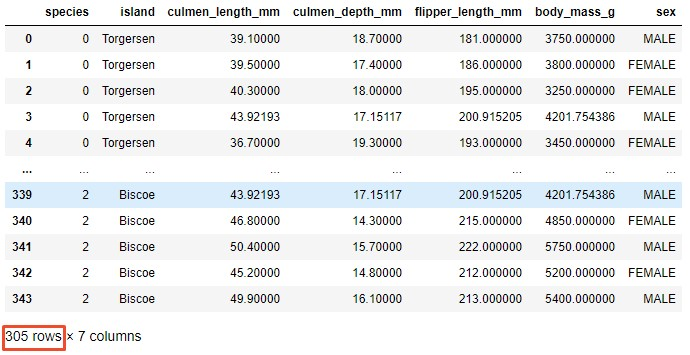After this operation, we have 305 samples in our dataset. With this approach we need to be extremely careful since it reduces the dataset size and highly depends on the data distribution.

## 4. Binning

Binning is a simple technique that groups different values into bins. For example, when we want to bin numerical features that would look like something like this:

• 0-10 – Low
• 10-50 – Medium
• 50-100 – High

In this particular case, we replace numerical features with categorical ones.However, we can bin categorical values too. For example, we can bin countries by the continent it is on:

• Serbia – Europe
• Germany – Europe
• Japan – Asia
• China – Asia
• USA – North America

The problem with binning is that it can downgrade performance, but it can prevent overfitting and increase the robustness of the machine learning model. Here is what that looks like in the code:## 5. Scaling

In previous articles, we often had a chance to how scaling helps machine learning models make better predictions. Scaling is done for one simple reason, if features are not in the same range, they will be treated differently by the machine learning algorithm. To put it in lame terms, if we have one feature that has a range of values from 0-10 and another 0-100, a machine learning algorithm might deduce that the second feature is more important than the first one just because it has a higher value. As we already know that is not always the case. On the other hand, it is unrealistic to expect that real data comes in the same range. That is why we use scaling, to put our numerical features into the same range. This standardization of data is a common requirement for many machine learning algorithms. Some of them even require that features look like standard normally distributed data. There are several ways we can scale and standardize the data, but before we go through them, let’s observe one feature of PalmerPenguins dataset ‘body_mass_g‘.

Also, observe the distribution of this feature:First, let’s explore scaling techniques that preserve distribution.

### 5.1 Standard Scaling

This type of scaling removes mean and scale data to unit variance. It is defined by the formula:where mean is the mean of the training samples, and std is the standard deviation of the training samples. The best way to understand it is to look at it in practice. For that we use SciKit Learn and StandardScaler class:We can see that the original distribution of data is preserved. However, now data is in range -3 to 3.

### 5.2 Min-Max Scaling (Normalization)

The most popular scaling technique is normalization (also called min-max normalization and min-max scaling). It scales all data in the 0 to 1 range. This technique is defined by the formula:If we use MinMaxScaler from SciKit learn library: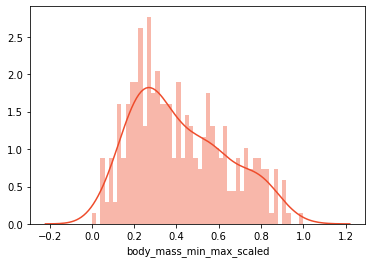Distribution is perserved, but data is now in range from 0 to 1.

### 5.3 Quantile Transformation

As we mentioned, sometimes machine learning algorithms require that the distribution of our data is uniform or normal. We can achieve that using QuantileTransformer class from SciKit Learn. First, here is how it looks like when we transform our data to uniform distribution:Here is the code that puts your data into normal distribution: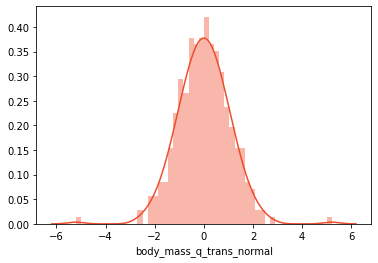Essentially, we use the output_distribution parameter in the constructor to define the type of distribution. Finally, we can observe scaled values of all features, with different types of scaling:## 6. Log Transform

One of the most popular mathematical transformations of data is logarithm transformation. Essentially, we just apply the log function to the current values. It is important to note that data must be positive, so if you need a scale or normalize data beforehand. This transformation brings many benefits. One of them is that the distribution of the data becomes more normal. In turn, this helps us to handle skewed data and decreases the impact of the outliers. Here is what that looks like in the code: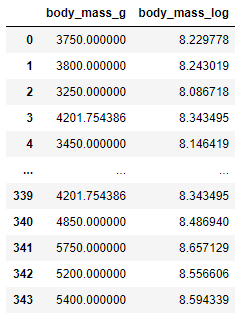If we check the distribution of non-transformed data and transformed data we can see that transformed data is closer to the normal distribution: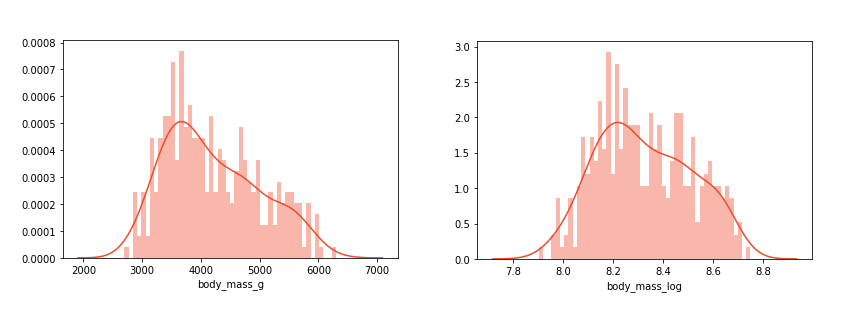## 7. Feature Selection

Datasets that are coming from the client are often huge. We can have hundreds or even thousands of features. Especially if we perform some of the techniques from above. A large number of features can lead to overfitting. Apart from that, optimizing hyperparameters and training algorithms, in general, will take longer. That is why we want to pick the most relevant features from the beginning.There are several techniques when it comes to feature selection, however, in this tutorial, we cover only the simplest one (and the most often used) – Univariate Feature Selection. This method is based on univariate statistical tests. It calculates how strongly the output feature depends on each feature from the dataset using statistical tests (like χ2). In this example, we utilize SelectKBest which has several options when it comes to used statistical tests (the default however is χ2 and we use that one in this example). Here is how we can do it:

Using hyperparameter k we defined that we want to keep the 3 most influential features from the dataset. The output of this operation is NumPy array which contains selected features. To make it into pandas Dataframe we need to do the following: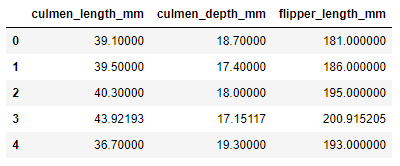## 8. Feature Grouping

The dataset that we observed so far is an almost perfect situation when it comes to terms of so-called “tidiness“. This means that each feature has it’s own column, each observation is a row, and each type of observational unit is a table. However, sometimes we have observations that are spread over several rows. The goal of the Feature Grouping is to connect these rows into a single one and then use those aggregated rows. The main question when doing so is which type of aggregation function will be applied to features. This is especially complicated for categorical features.

As we mentioned, PalmerPenguins dataset is very tydi so the following example is just educational to show the code that can be used for this operation:

Here we grouped data by spicies value and for each numerical value we created two new features with sum and mean value.## 9. Feature Split

Sometimes, data is not connected over rows, but over colums. For example, imagine you have list of names in one of the features:

So, if we want to extract only first name from this feature we can do the following:

This technique is called feature spliting and it is often used with string data.

## Conclusion

In this article, we had a chance to explore the 9 most commonly used techniques for feature engineering. As a bonus, here is the recording of Benedict mispronounced the word “penguins” (and making me misspelling that word a dozen times during the creation of this article).#### Nikola M. Zivkovic

CAIO at Rubik's Code

Nikola M. Zivkovic a CAIO at Rubik’s Code and the author of book “Deep Learning for Programmers“. He is loves knowledge sharing, and he is experienced speaker. You can find him speaking at meetups, conferences and as a guest lecturer at the University of Novi Sad.

Rubik’s Code is a boutique data science and software service company with more than 10 years of experience in Machine Learning, Artificial Intelligence & Software development. Check out the services we provide.

Ultimate Guide to Machine Learning with Python

This bundle of e-books is specially crafted for beginners.

Everything from Python basics to the deployment of Machine Learning algorithms to production in one place.

Become a Machine Learning Superhero TODAY!